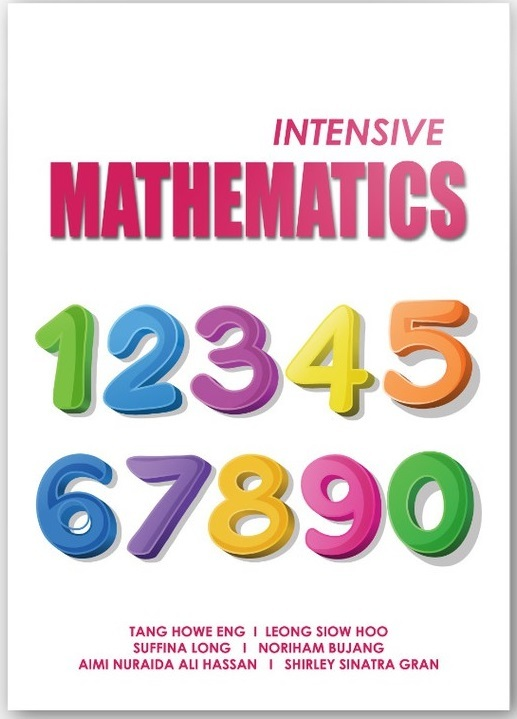•# Intensive Mathematics

Regular price
RM 40.00
Sale price
RM 40.00
Regular price
RM 0.00

Addressing the importance of fundamental mathematics for further study in the fields require mathematical knowledge and skills, the intensive Mathematics introduces concepts from fundamental definitions of algebra and statistics as well as the theories and applications. The book aims to help readers intensively develop the fundamental knowledge of mathematics. It begins with prominent topic used in solving mathematical problems such as number and operation, algebraic expression, equation, function, index and logarithm. Once the foundation is established, subsequent topics required problem solving and applications are introduced such as sequence, application in business mathematics and statistics.

Throughout each chapter the concepts have been arranged from the simplest to more complicated and are explained in a comprehensible way. Relevant examples and exercise with answers are also provided intensive Mathematics is an excellent book for students and instruction.